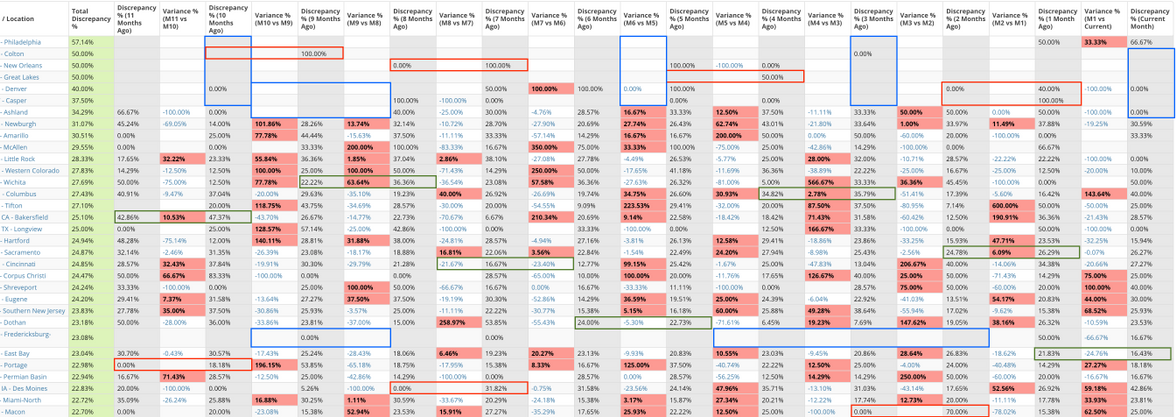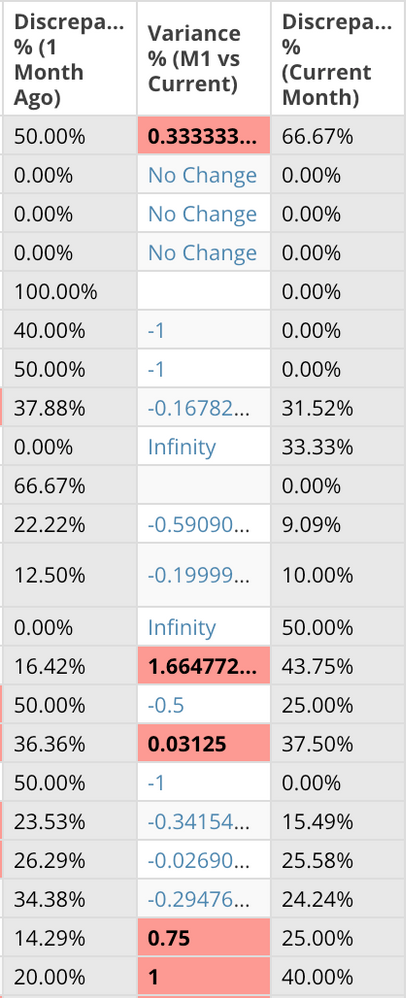# Beast Mode assistance (issues w/month-over-month calculations for 12 month table)

This is a followup to a thread from last month (Table, compare several month-over-month). We've created a table card that for the most part function as requested, but noticed a few things that need to be accounted for and we haven't been able to figure those out at this time. In the screenshot below, we have a branch/location and the Total Discrepancies for each over the last 12 months; then the table will show, from left to right, the Monthly Discrepancy% followed by the Variance % when compared to the next Monthly Discrepancy%. We created a beast mode for each column, and as stated these are functional and provide exactly what the request was looking for (green boxes show some examples of this, and percentage increases were conditionally formatted to have the cell in red).

However, some of the issues we see with the table, i.e. the beast modes themselves, are as follows (screenshot will show each of these as they appear when viewing the card):

1) for Monthly Discrepancies where no discrepancies exist, for some reason some boxes show 0% while others are BLANK

2) since going from 0% to any other percentage is infinite, those fields are also BLANK (want those to populate INFINITE in red (hoping we can do this in CHART PROPERTIES/COLORS, but may be able to add HTML/CSS to the existing beast mode if not)

3) lastly, we'd like to get clarification on whether or not we should leave all of the new columns as beast modes (currently 23 in total), or if it'd be better doing these in the dataflow itself by way of transform. Not sure if that would slow down the dataflow, but we did notice the card can take 5-10 seconds to load (not a major concern and likely due to the number of beast modes being calculated).Discrepancy% Beast Mode:

(CASE
WHEN SUM(`Has Discrepancy`) = '0' OR COUNT(`OfferId`) = '0' THEN 0 ELSE

SUM(CASE WHEN MONTH(`ReviewedDate`) = MONTH(CURRENT_DATE()) THEN `Has Discrepancy`
END)

/
COUNT(CASE WHEN MONTH(`ReviewedDate`) = MONTH(CURRENT_DATE()) THEN `ID#`
END)
END)

Variance% Beast Mode:

(CASE
WHEN SUM(`Has Discrepancy`) = '0' OR COUNT(`ID#`) = '0' THEN 0 ELSE

SUM(CASE WHEN MONTH(`ReviewedDate`) = MONTH(CURRENT_DATE()) THEN `Has Discrepancy`
END)

COUNT(CASE WHEN MONTH(`ReviewedDate`) = MONTH(CURRENT_DATE()) THEN `ID#`
END)
END)

(CASE
WHEN SUM(`Has Discrepancy`) = '0' OR COUNT(`ID#`) = '0' THEN 0 ELSE
SUM(CASE WHEN MONTH(DATE_ADD(`ReviewedDate`,INTERVAL 1 MONTH)) = MONTH(CURRENT_DATE()) THEN `Has Discrepancy`
END)

/
COUNT(CASE WHEN MONTH(DATE_ADD(`ReviewedDate`,INTERVAL 1 MONTH)) = MONTH(CURRENT_DATE()) THEN `ID#`
END)
END) - 1.00

Thanks in advance for any assistance provided...

John

•CoachI believe that the reason the beastmode is not calculating is because there was either an error in the calculation (divide by 0) or the case statement was not fulfilled (in this case, is it possible for a record not have a `Has Discrepancy` value?)

You may want to try something like this to make sure that the case statement calculates for all rows of data:

CASE WHEN COUNT(CASE WHEN MONTH(`ReviewedDate`)= MONTH(CURRENT_DATE()) THEN `ID#` END)=0 THEN 0 ELSE
(CASE
WHEN SUM(IFNULL(`Has Discrepancy`,0)) = '0' OR COUNT(`OfferId`) = '0' THEN 0 ELSE
SUM(CASE WHEN MONTH(`ReviewedDate`) = MONTH(CURRENT_DATE()) THEN `Has Discrepancy`
END)

/
COUNT(CASE WHEN MONTH(`ReviewedDate`) = MONTH(CURRENT_DATE()) THEN `ID#`
END)
END)
END

“There is a superhero in all of us, we just need the courage to put on the cape.” -Superman

•CoachI believe that the reason the beastmode is not calculating is because there was either an error in the calculation (divide by 0) or the case statement was not fulfilled (in this case, is it possible for a record not have a `Has Discrepancy` value?)

You may want to try something like this to make sure that the case statement calculates for all rows of data:

CASE WHEN COUNT(CASE WHEN MONTH(`ReviewedDate`)= MONTH(CURRENT_DATE()) THEN `ID#` END)=0 THEN 0 ELSE
(CASE
WHEN SUM(IFNULL(`Has Discrepancy`,0)) = '0' OR COUNT(`OfferId`) = '0' THEN 0 ELSE
SUM(CASE WHEN MONTH(`ReviewedDate`) = MONTH(CURRENT_DATE()) THEN `Has Discrepancy`
END)

/
COUNT(CASE WHEN MONTH(`ReviewedDate`) = MONTH(CURRENT_DATE()) THEN `ID#`
END)
END)
END

“There is a superhero in all of us, we just need the courage to put on the cape.” -Superman
•CoachIf that works, then you can just add similar logic to the variance beast mode.  If not, it would be helpful to see some sample data to better understand what your data set looks like.

To answer your questions about performance... In my experience, adding these kinds of calculations to a dataflow has minimal impact on performance.  Dataflow performance tends to get bogged down when doing complicated joins or not indexing properly.  Simple calculations like this should not slow things down much, if at all.

The advantage to keeping the calculation as a beastmode is that it will adjust based on the filters you apply to the card.  For example, if you wanted to focus on only the Northwest, you could filter the card and all of the calculations would reflect the change.  If you perform the calcuations in the data flow, then you will only be able to view the field the way that it is being calculated in the data flow.

Hope that helps.  Let me know if you have more questions.

“There is a superhero in all of us, we just need the courage to put on the cape.” -Superman
• @ST_-Superman-_, thanks for the reply. We added a case statement to the dataflow via transform so that "Has Discrepancy" will always be populated as it corresponds to the ID# (1 for a discrepancy, or 0 when no discrepancies exist).

Initially, forgot to update the outer case statement, i.e. MONTH(DATE_ADD) to reflect the correct month, but once we updated that for each beast mode the correction you provided works and all discrepancy% fields are now populated (with either an actual discrepancy% or 0%).

CASE WHEN COUNT(CASE WHEN MONTH(DATE_ADD(`ReviewedDate`,INTERVAL 1 MONTH)) = MONTH(CURRENT_DATE())

For the variance, we've tried several combinations based on your reply but still haven't been able to get it to populate for all months (the expectation was that it would at least populate whenboth months, prior and after, were  0% by displaying a variance of 0% but it didn't).

That said, once we are able to get this corrected, is there a way to show a text string as opposed to a numeric value for any fields where (seem to recall reading in some cases this is not an option):

1) Variance = 0 (from 0% to 0%): N/A, NaN, or No Change

2) Variance = INFINITE (from 0% to XX%): Infinite or something along those lines

P.S. - I can share some sample data if it's ok to DM you with an Excel file, or any other format that you might prefer?

•CoachI think it would be easiest if I could get a few rows of sample data.

There is a problem with changing the output from a value to a text string.  Domo needs to set a value type for each field.  If you were to write a case statement like:

case when `A` - `B` = 0 then 'NaN' else `A` - `B` end

then the beastmode would error out because 'NaN' is a text field and `A`-`B` would be a value.

You could do something like

case when `A` - `B` = 0 then 'NaN' else concat(`A` - `B`) end

This would result in a text field.

This doesn't work quite as well for the variance field because format wise, 57% would become 0.57

If you want to DM me some sample data I will do my best to help out

“There is a superhero in all of us, we just need the courage to put on the cape.” -Superman
• @ST_-Superman-_ yeah, just found that out (screenshot shows outcome of changing the beast mode similar to what you outlined - adds No Change to some cells and others NaN, but also populates Infinite which is a good thing though it also changes the %'s)!

I'll DM you some sample data, thanks for your help on this!•CoachYou could also do

concat(round((`Month 1` / `Month 2`)*100,1),'%')

replace `Month 1` / `Month 2` with the actual calculation.  but that would give you something like this for the format 33.3% or 75.0%

It would be a text field though, so you couldn't do any more calculations with it.

“There is a superhero in all of us, we just need the courage to put on the cape.” -Superman